# Project Definition

## Project Overview

Analyzing and classifying images is a common approach in the machine learning problem space, and has countless real-world applications that can be seen everyday and everywhere. One such example is quickly reading and classifying handwritten zipcode digits in the postal system. This project utilizes the zipcode dataset — commonly found in machine learning and data mining literature — to explore the application of linear regression and K Nearest Neighbors (KNN) for classifying handwritten digits (1). Because this dataset is notoriously difficult (typically a 2.5% error rate is considered excellent), the problem-space was subsetted to only include “2” and “7” digits. These two were considered similar enough to still provide a challenge to the model.

## Problem Statement

The goal is to classify examples of handwritten digits as accurately as possible; the tasks involved in achieving this are the following:

1. Download and explore the data; preprocess if necessary
2. Train classifiers that can determine if a number is either a “2” or a “7”
3. Evaluate initial model performance
4. Refine models with cross-validation for parameter selection
5. Evaluate final model performance on testing dataset

The final model is expected to be accurate and quick enough for implementation in a system such as the postal service.

## Metrics

Accuracy will be used to measure the effectiveness of the classification models built. In this instance, cut-offs were used with the linear regression model to provide a discrete result instead of continuous, and the KNN model likewise provides a discrete result.

Accuracy is a common metric when using binary classifiers since it equally weights the true positives and true negatives, and provides a clear communication of correctness. Accuracy is defined as follows:

# Analysis

## Data Exploration

The zipcode dataset contains normalized handwritten digits, automatically scanned from envelopes by the U.S. Postal Service. The original digits were of different sizes and orientations and were binary. After normalizing and deslanting, 16x16 greyscale images were produced. The dataset contains all digits, 0–9, in the following distributions and proportions: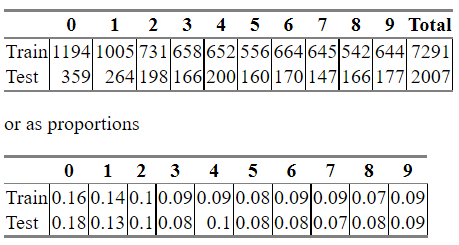Zipcode dataset values and their distributions. (1)

The training set contains 7291 observations, while the test set has 2007 observations. After filtering to only rows where the response variable is either a “2” or a “7”, 1376 rows result. The number of columns — 257 — did not change, as our filtering technique only addressed the number of observations.

Counting the number of 2’s and 7’s gives a simple summary statistic. We would hope that these would be roughly equal, and we see that they are. There are 731 “2s” (53%) in the dataset, and 645 “7s”(47%).

There were no significant abnormalities in the dataset, as it was normalized prior to consumption.

## Data Visualization

To visualize the dataset, we can reshape a row into a 16x16 matrix and plot it as an image. We can see that each one differs in appearance and shape. Several examples of “2s” and “7s” are shown below. While sometimes a number is easily identifiable, other times it requires interpretation.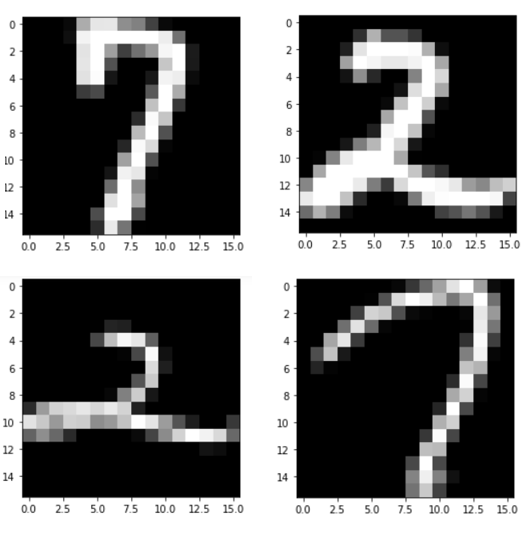Examples of “2” and “7” data.

# Methodology

## Data Preprocessing

No extensive preprocessing was needed for this dataset, as it had been completed prior to its distribution. As noted above, the original digits were binary and of many different sizes and orientations. They were then deslanted and normalized to produce the 16x16 greyscale images seen in the final public dataset.

The dataset was subsetted to include only rows where the response variable, was either a “2” or a “7”, and all other rows were removed.

## Implementation

The implementation process can be summarized into the following steps:

1. Linear regression & KNN classifier training stage
2. Model parameter refinement
3. Verify linear regression and KNN model performance on test data
4. Evaluate models using Monte Carlo cross-validation

Each of the classifiers — linear regression and KNN — were trained on the preprocessed training data and then tested on the testing data. The linear regression model was run with default parameters, and the KNN model was run with varying values of k — ranging from 1 to 15, in step sizes of 2.

## Refinement

Both the linear regression and KNN models were refined for their parameters and methods.

Linear Regression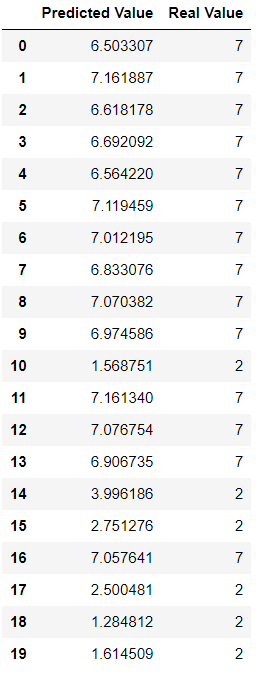Initial linear regression predicted values and their corresponding true values. Collected on training data.

Because linear regression outputs a continuous prediction, it is no surprise that the initial accuracy of the model was quite low, since it only counts exact matches and the outputs included values other than 2 and 7. The image to the left shows some examples of predicted values that are often very close to the true value, but were not counted as such in the accuracy metric.

To account for this, the predicted results were rounded, with any value greater than or equal to a 4.5 being rounded up to a 7, and anything less than a 4.5 being rounded down to a 2. This drastically improved the model’s performance, as can be seen below.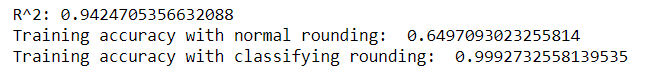Linear regression model performance on training data.

Since the dataset is of relatively small size, cross-validation was done in order to verify the performance of the model. The training and testing dataset were combined into one full set, with 1721 records, and on each of the 100 cross-validation runs the data was randomly split into new training and testing sets using an 80/20 split.

KNN

In order to optimize the performance of the KNN model, the model parameter k was tested using eight different values: [1, 3, 5, 7, 9, 11, 13, 15].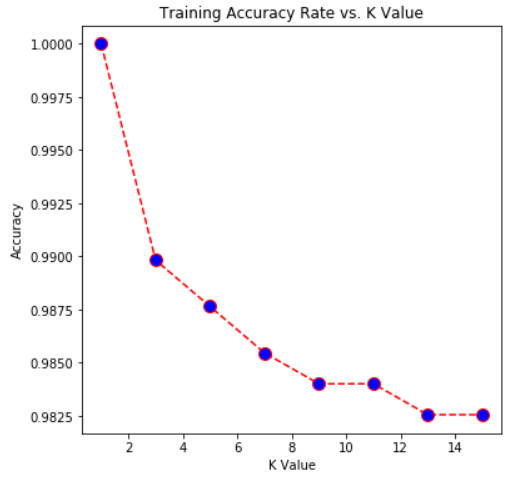KNN model performance on training data.

These results indicate that the performance continually degrades as more and more neighbors are taken into account. However, though the curve is steep, the accuracy values are still very high — with the lowest value appearing on the chart being a 0.9825.

Furthermore, cross-validation was used in order to verify the performance of the KNN model. The full 1721 record dataset was used, and was randomly split into new training and testing sets using an 80/20 split. Cross-validation was performed 100 times using each of the k values listed above. Below are the resulting means and variances of the errors for each value of k.

# Results

## Model Evaluation & Validation

Both models were validated on the testing dataset. The linear regression model performed about the same on the testing data as on the training data — with only a ~0.004 difference in the rounded accuracy score. Usually the testing performance is worse than the training, but in this instance the results are nearly indistinguishable.

The KNN model and its different k values was also appled to the testing dataset. Here we can see distinguishably lower model accuracies, as expected, and a different result as far as which k vales produced the highest accuracy. In the training data, k=1 gave 100% accuracy and k=3 yielded a 99% accuracy. When applied to the testing data, the models with k=3 and k=5 performed best, both with accuracies of ovr 98.5%.

A summary of the two classifer models and their performance on the training and testing data is shown below.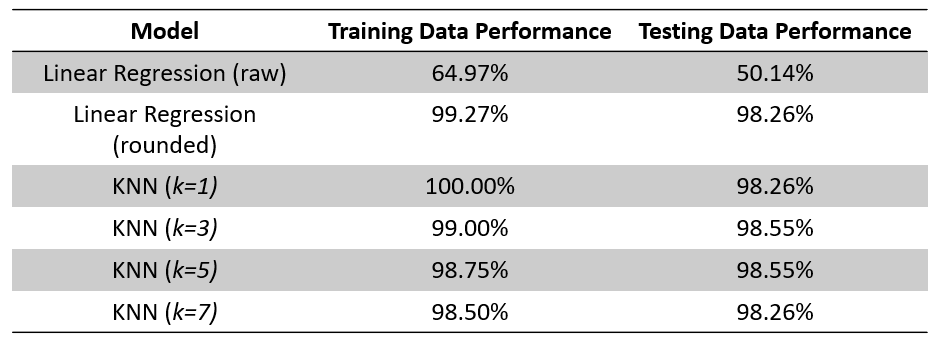Summary of model performances on training and testing data.

## Justification

When the models were evaluated on only the training datasets, they had understandably higher performances than on the testing dataset. However, a better performance in this instance does not mean a better model, as the model was overfitted to the data and would never perform equally on another set of data. This can be seen with both the linear regression model and the KNN model. Additionally, the graph (shown above) illustrating the KNN model accuracies on the training data strongly resembles a logarithmic curve — with each new accuracy decreasing slightly until it begins to level off. In reality, these errors should be a bit more random.

When fitting the linear regression model with the rounding approach, two different methods were used to classify the outcomes and thus evaluate the model performance. First, the outputs were rounded to integers and then compared for equality. This resulted in values such as 4, 5, 6, and 8 being included and the overall model performance to be very low — an error of 0.35. However, a second approach (the rounded approach shown and discussed above) was used to remedy this and account for the discrete nature of the data. Using the model predictions, if a value was greater than or equal to 4.5 then it was classified as a 7, and if less than 4.5 it was classified as a 2.

Monte Carlo cross validation was used to provide additional confidence in the models’ results. For each model, 100 iterations were conducted and their results averaged. The linear regression model produced a model error of 0.0116 and model variance of 3.20e-05 — both indicating excellent performance. Likewise, for each value of k, 100 iterations were run and averaged to find the model error and variation. Wecan see that k=1 and k=3 are the best performing models, a slight difference from the previous results only using one run of testing data for evaluation. k=3 slightly outperformed the k=1 model, but both were incredibly close and nearly indistinguishable in result.

# Conclusion

## Reflection

Accurately classifying handwritten digits can be achieved using either a linear regression or a KNN classifier model, as both were found to perform well on this dataset.

However, when looking only at the KNN models, it can be seen that the optimal tuning parameter for k is k=3. This model has the lowest error and only a slightly higher variance than the k=1 model. Because k=3 also performed dependably using only one test dataset, we choose k=3 as our optimal tuning parameter.

Something interesting to consider would be to see if a model could accurately identify all digits from the dataset, instead of just a set of two. This multi-output regression problem would likely yield much lower accuracies, but is a more realistic problem faced in the real world. I found the applicability of this project to be the most interesting aspect for me, since many data science projects and problems are usually hypothetical or involved with a dataset you don’t normally encounter in your everyday life.

## Improvement

Because only two types of models were trained and compared on this dataset, additional types could be explored to see if performance varied. Because both the linear regression and KNN models had similar performance, other models most likely would perform similarly as well.

Instead of a linear regression model with imposed cutoffs to handle the discrete nature of the data, a logistic regression model could have been run in its place. Or, instead of using a clean split in the values between 2 and 7 (<4.5 = 2, ≥4.5=7) I could have removed the middle portion and forced “correct” results to be closer to their true value. This would likely reduce model accuracy, but would provide a clearer look at model performance as the first set of cutoffs was quite generous.

# Citations

(1) US Post Office Zip Code Data. Stanford University. (n.d.). https://web.stanford.edu/~hastie/StatLearnSparsity_files/DATA/zipcode.html.

--

--